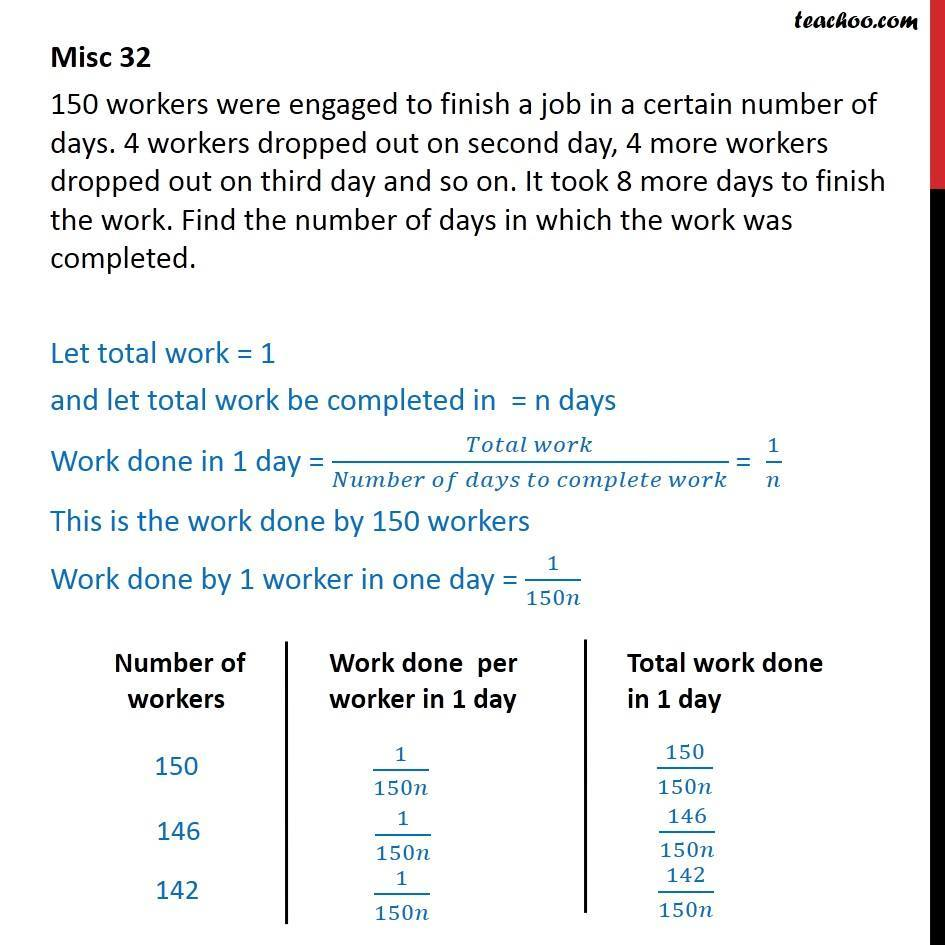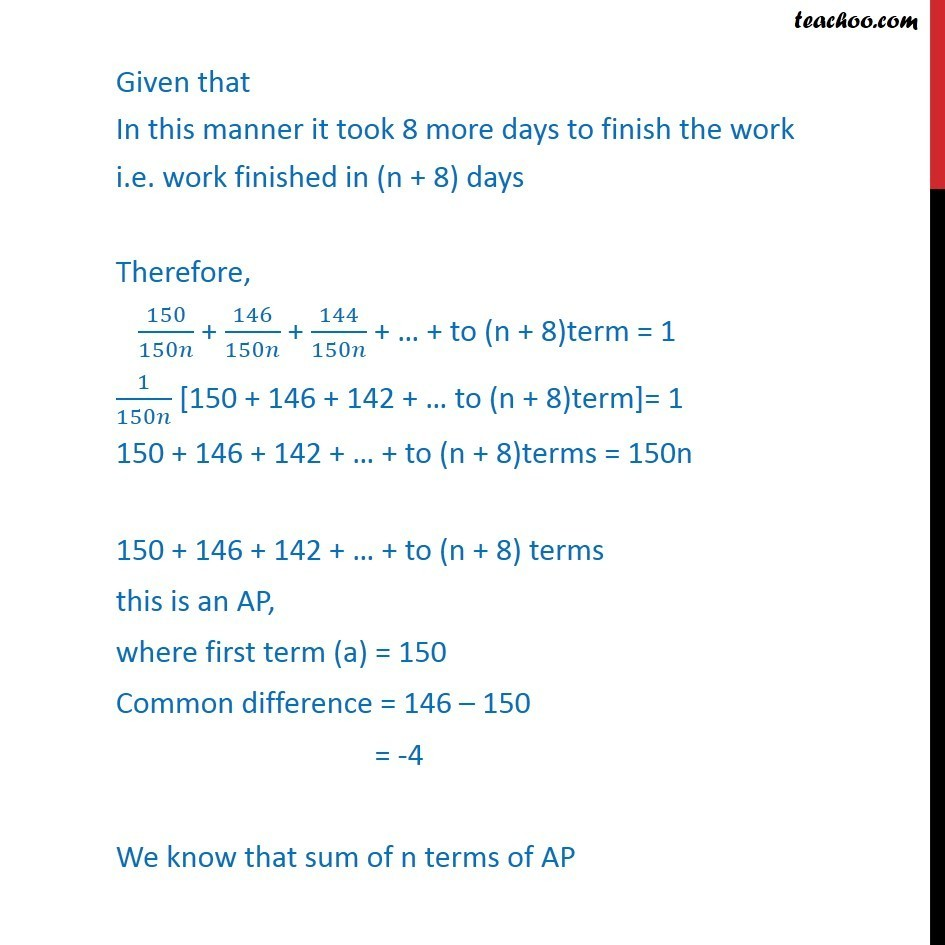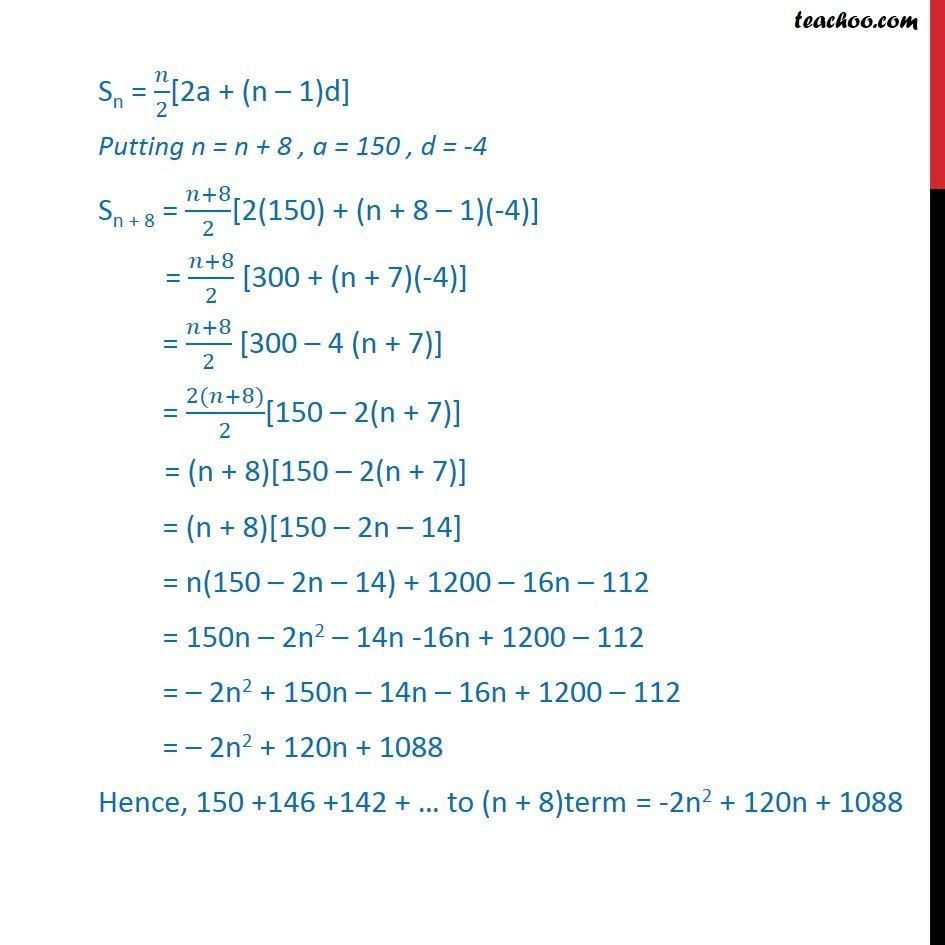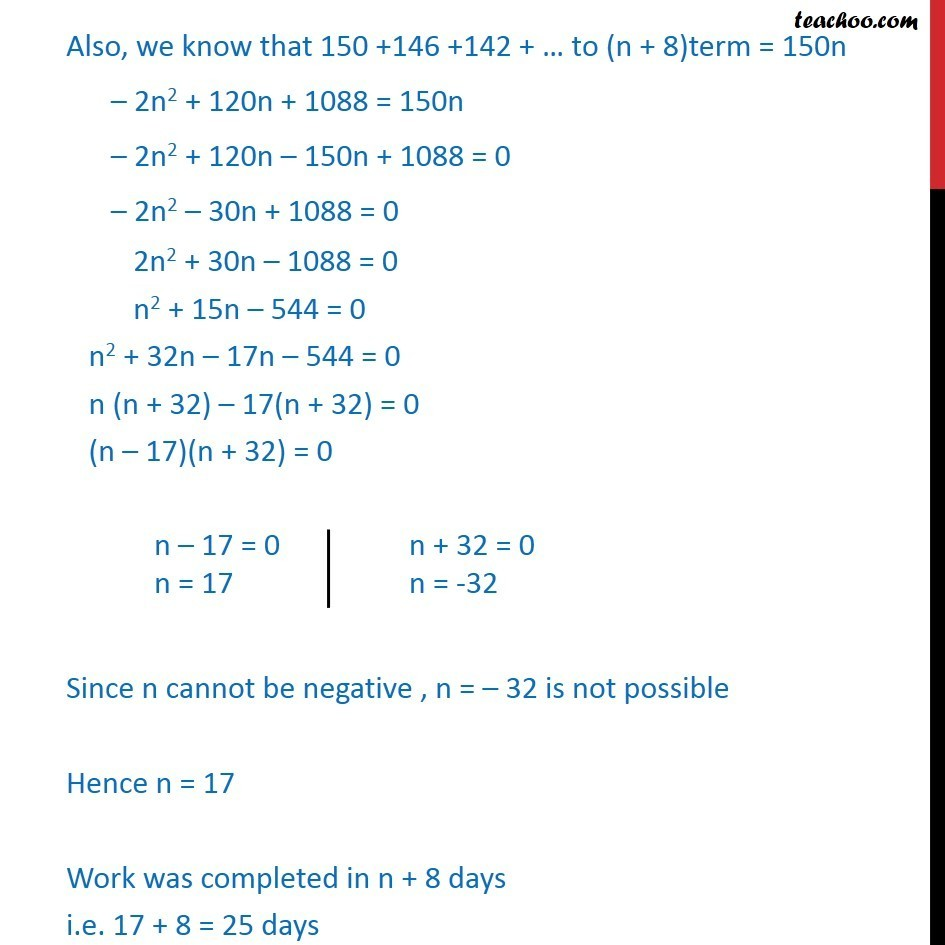Finding sum from nth number

Chapter 8 Class 11 Sequences and Series
Concept wiseLearn in your speed, with individual attention - Teachoo Maths 1-on-1 Class

### Transcript

Misc 18 150 workers were engaged to finish a job in a certain number of days. 4 workers dropped out on second day, 4 more workers dropped out on third day and so on. It took 8 more days to finish the work. Find the number of days in which the work was completed. Let total work = 1 and let total work be completed in = n days Work done in 1 day = (𝑇𝑜𝑡𝑎𝑙 𝑤𝑜𝑟𝑘)/(𝑁𝑢𝑚𝑏𝑒𝑟 𝑜𝑓 𝑑𝑎𝑦𝑠 𝑡𝑜 𝑐𝑜𝑚𝑝𝑙𝑒𝑡𝑒 𝑤𝑜𝑟𝑘) = 1/𝑛 This is the work done by 150 workers Work done by 1 worker in one day = 1/150𝑛 Given that In this manner it took 8 more days to finish the work i.e. work finished in (n + 8) days Therefore, 150/150𝑛 + 146/150𝑛 + 144/150𝑛 + … + to (n + 8)term = 1 1/150𝑛 [150 + 146 + 142 + … to (n + 8)term]= 1 150 + 146 + 142 + … + to (n + 8)terms = 150n 150 + 146 + 142 + … + to (n + 8) terms this is an AP, where first term (a) = 150 Common difference = 146 – 150 = -4 We know that sum of n terms of AP Sn = 𝑛/2[2a + (n – 1)d] Putting n = n + 8 , a = 150 , d = -4 Sn + 8 = (𝑛+8)/2[2(150) + (n + 8 – 1)(-4)] = (𝑛+8)/2 [300 + (n + 7)(-4)] = (𝑛+8)/2 [300 – 4 (n + 7)] = (2(𝑛+8))/2[150 – 2(n + 7)] = (n + 8)[150 – 2(n + 7)] = (n + 8)[150 – 2n – 14] = n(150 – 2n – 14) + 1200 – 16n – 112 = 150n – 2n2 – 14n -16n + 1200 – 112 = – 2n2 + 150n – 14n – 16n + 1200 – 112 = – 2n2 + 120n + 1088 Hence, 150 +146 +142 + … to (n + 8)term = -2n2 + 120n + 1088 Also, we know that 150 +146 +142 + … to (n + 8)term = 150n – 2n2 + 120n + 1088 = 150n – 2n2 + 120n – 150n + 1088 = 0 – 2n2 – 30n + 1088 = 0 2n2 + 30n – 1088 = 0 n2 + 15n – 544 = 0 n2 + 32n – 17n – 544 = 0 n (n + 32) – 17(n + 32) = 0 (n – 17)(n + 32) = 0 Since n cannot be negative , n = – 32 is not possible Hence n = 17 Work was completed in n + 8 days i.e. 17 + 8 = 25 days